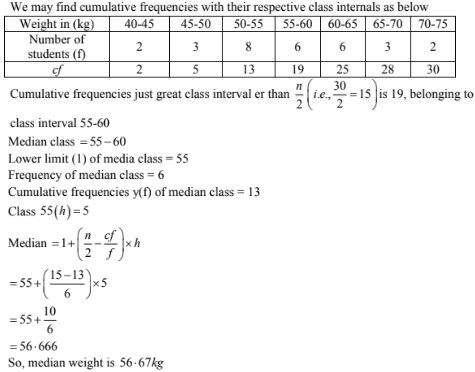# RD Sharma Solutions Chapter 7 Statistics Exercise 7.4 Class 10 Maths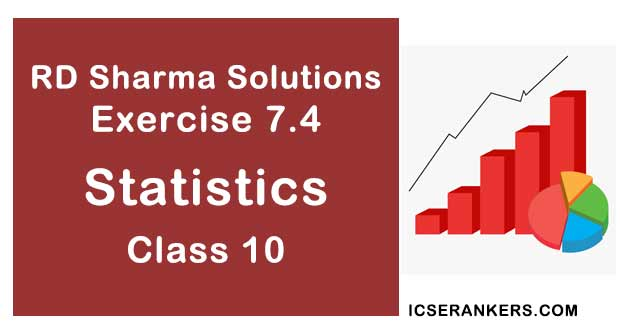Chapter Name RD Sharma Chapter 7 Statistics Book Name RD Sharma Mathematics for Class 10 Other Exercises Exercise 7.1Exercise 7.2Exercise 7.3Exercise 7.5Exercise 7.6 Related Study NCERT Solutions for Class 10 Maths

### Exercise 7.4 Solutions

1. Following are the lives in hours of 15 pieces of the components of aircraft engine. find the median:

715,724,,725,710,729,745,694,699,696,712,734,728,716,705,719.

Solution

Lives in hours of is pieces are
= 715, 724, 725, 710, 729, 745, 694, 699, 696, 712,734,728,719,705,705,719
Arrange the above data in a sending order
694,696,699,705,710,712,715,716,719,721,725,728,729,734,745
N = 15(odd)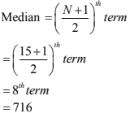2. The following is the distribution of height of students of a certain class in a certain city.

 Height (in cm): 160 – 162 163 – 165 166 – 168 169 – 171 172 – 174 No. of students: 15 118 142 127 18

Find the median height.

Solution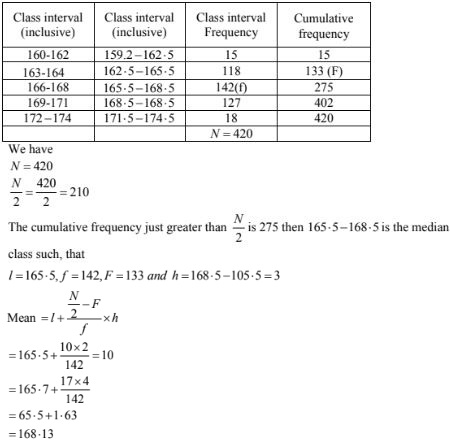3. Following is the distribution of I.Q. of loo students. Find the median I.Q.
 I.Q.: 55 – 64 65 – 74 75 – 84 85 – 94 95 – 104 105 – 114 115 – 124 125 – 134 135 – 144 No. of Students: 1 2 9 22 33 22 8 2 1

Solution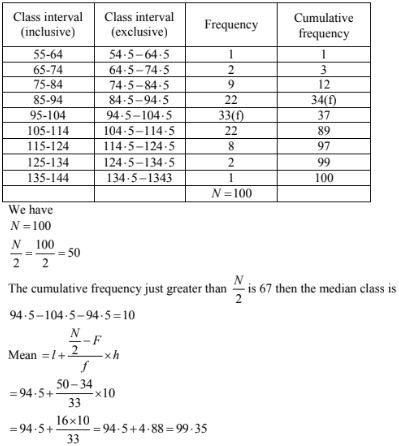4. Calculate the median from the following data:
 Rent (in Rs.): 15 – 25 25 – 35 35 – 45 45 – 55 55 – 65 65 – 75 75 – 85 85 – 95 No. of houses: 8 10 15 25 40 20 15 7

Solution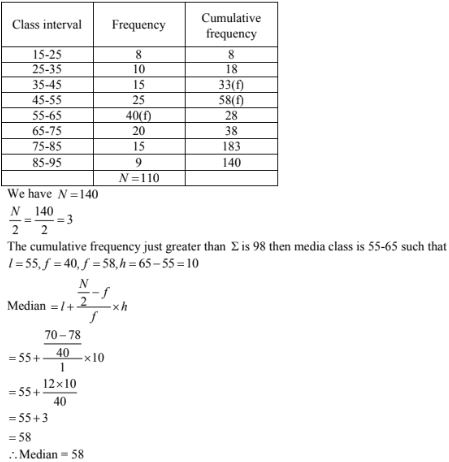5. Calculate the median from the following data:
 Marks below: 10 20 30 40 50 60 70 80 No. of students: 15 35 60 84 96 127 198 250

Solution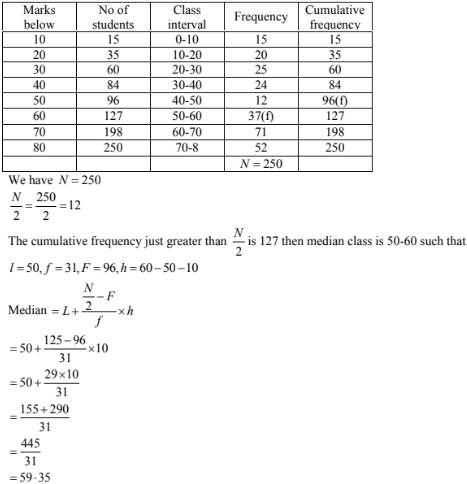6. An incomplete distribution is given as follows:
 Variable: 0 – 10 10 – 20 20 – 30 30 – 40 40 – 50 50 – 60 60 – 70 Frequency: 10 20 ? 40 ? 25 15

Solution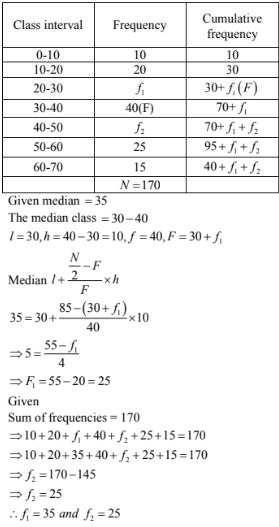7. Calculate the missing frequency from the following distribution, it being given that the median of the distribution is 24.
 Age in years: 0 – 10 10 – 20 20 – 30 30 – 40 40 – 50 No. of persons: 5 25 ? 18 7

Solution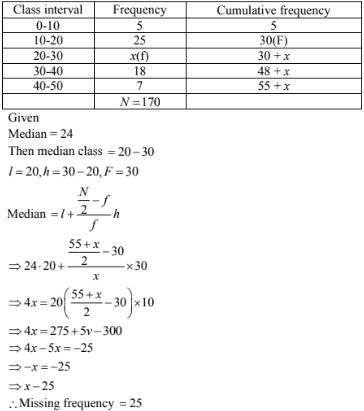8. Find the missing frequencies and the median for the following distribution if the mean is 1.46.
 No. of accidents: 0 1 2 3 4 5 Total Frequency (No. of days): 46 ? ? 25 10 5 200

Solution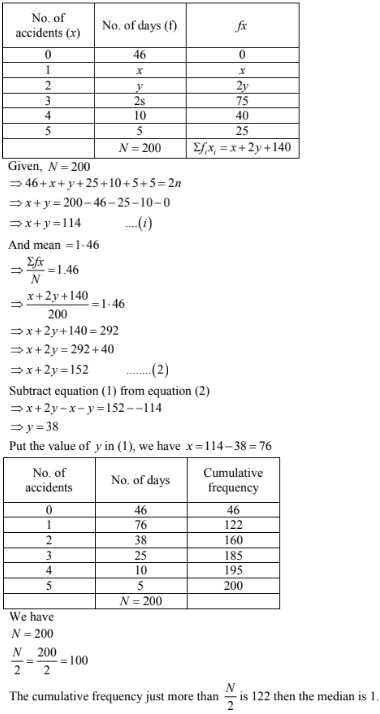9. An incomplete distribution is given below:
 Variable: 10 – 20 20 – 30 30 – 40 40 – 50 50 – 60 60 – 70 70 – 80 Frequency: 12 30 - 65 - 25 18

You are given that the median value is 46 and the total number of items is 230.
(i) Using the median formula fill up missing frequencies.
(ii) Calculate the AM of the completed distribution.

Solution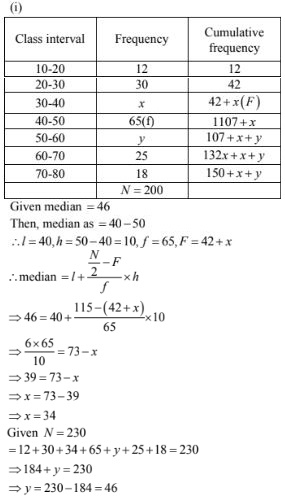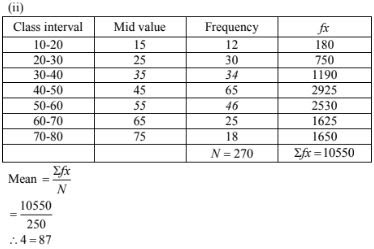10. The following table gives the frequency distribution of married women by age at marriege:
 Age(in years) Frequency Age(in years) Frequency 15 – 19 53 40 – 44 9 20 – 24 140 45 – 49 5 25 – 29 98 50 – 54 3 30 – 34 32 55 – 59 3 35 – 39 12 60 and above 2

Calculate the median and interpret the results

Solution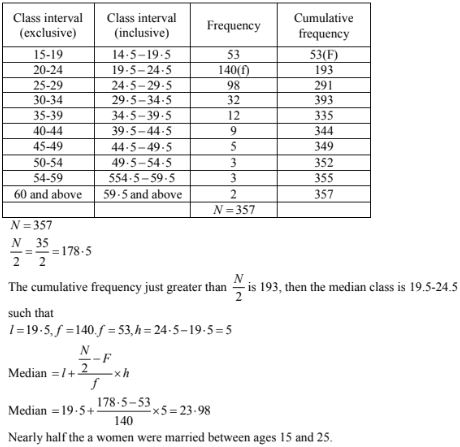11. If the median of the following frequency distribution is 28.5 find the missing frequencies:
 Class interval: 0 – 10 10 – 20 20 – 30 30 – 40 40 – 50 50 – 60 Total Frequency: 5 F1 20 15 F2 5 60

Solution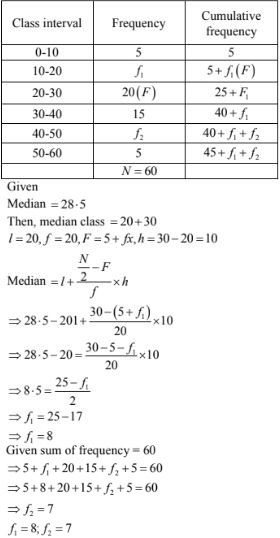12. The median of the following data is 525. Find the missing frequency, if it is given that there are 100 observations in the data:
 Class interval Frequency Class interval Frequency 0 – 100 2 500 – 600 20 100 – 200 5 600 – 700 F2 200 – 300 F1 700 – 800 9 300 – 400 12 800 – 900 7 400 – 500 17 900 – 1000 4

Solution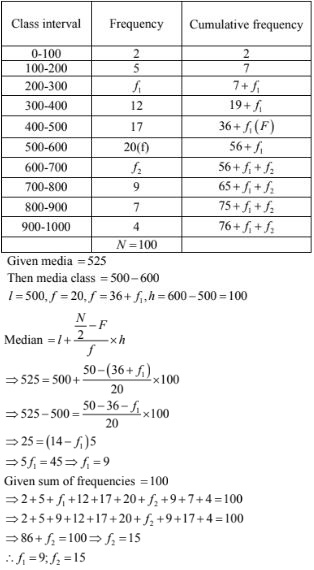13. If the median of the following data is 32.5, find the missing frequencies.
 Class interval: 0 – 10 10 – 20 20 – 30 30 – 40 40 – 50 50 – 60 60 – 70 Total Frequency: F1 5 9 12 F2 3 2 40

Solution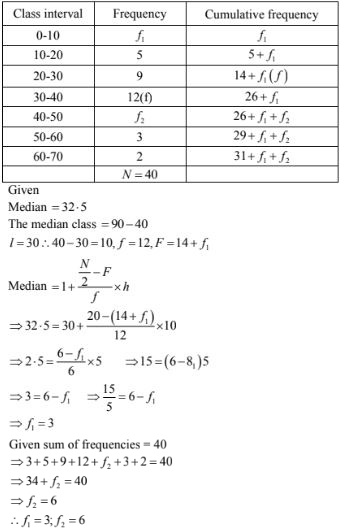14. A survey regarding the height(in cm) of 51 girls of X of a school was conducted and the following data was obtained:
 (i) Marks No. of students (ii) Marks No. of students Less than 10 0 More than 150 0 Less than 30 10 More than 140 12 Less than 50 25 More than 130 27 Less than 70 43 More than 120 60 Less than 90 65 More than 110 105 Less than 110 87 More than 100 124 Less than 130 96 More than 90 141 Less than 150 100 More than 80 150

Solution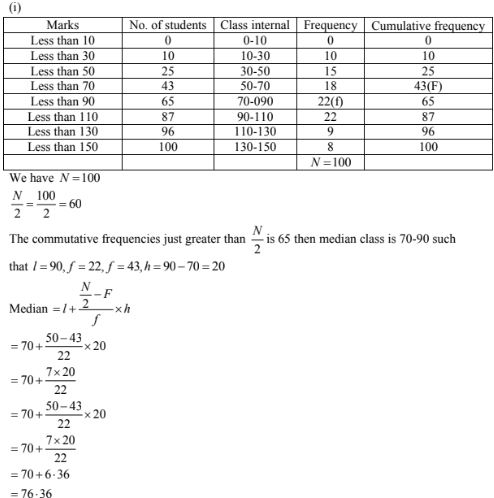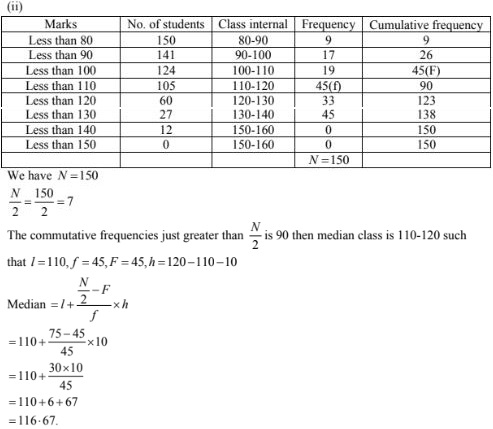15. A survey regarding the height(in cm) of 51 girls of class X of a school was conducted and the following data was obtained:
 Height in cm Number of Girls Less than 140 4 Less than 145 11 Less than 150 29 Less than 155 40 Less than 160 46 Less than 165 51

Find the median height.

Solution

To calculate the median height, we need to find the class intervals and their corresponding frequencies
The given distribution being of thee less than type 140, 145, 150,  ........, 165 give the upper limits of corresponding class intervals. So, the classes should be below 140 , 145, 150, .....160, 165  observe that from the given distribution , we find that there are 4 - girls with height less than 140 is 4. Now there are 4 girls with heights less than 140. Therefore, the number of girls with height in the interval 140, 145 is 11 - 4 = 7, similarly. The frequencies of 145, 150 is 29 - 11 = 18, for 150 - 155 it is 40 -29 =11, and so on so our frequencies distribution becomes.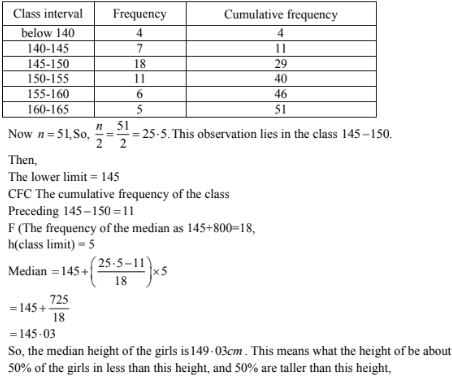16. A life insurance agent found the following data for distribution of ages of 100 policy holders. Calculate the median age, if policies are only given to persons having age 18 years onwards but less than 60 years.
 Age in years Number of policy holders Below 20 2 Below 25 6 Below 30 24 Below 35 45 Below 40 78 Bwlow 45 89 Below 50 90 Below 55 98 Below 60 100

Solution

Here class width is not same. There is no need to adjust the frequencies according to class intervals. Now given frequencies table is of less than type represented with upper class limits. As policies were given only to persons having age 18 years onwards but less than 60 years we can definite class intervals with their respective cumulative frequencies as below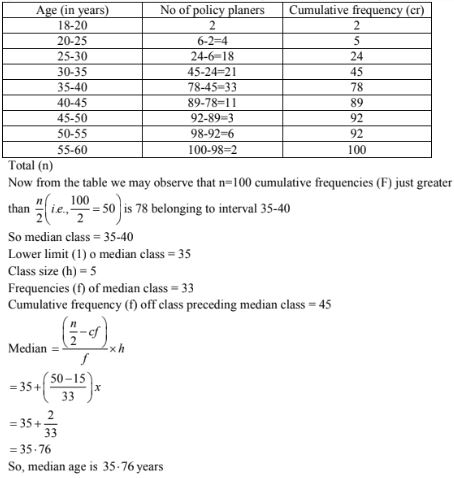17. The lengths of 40 leaves of a plant are measured correct to the nearest millimeter, and the data obtained is represented in the following table:
 Length( in mm): 118 – 126 127 – 135 136 – 144 145 – 153 154 – 162 163 – 171 172 – 180 No.of leaves: 3 5 9 12 5 4 2

Find the mean length of life.

Solution

The given data is not having continuous class intervals is 1. So, we have to add and subtract 1/2 = 0.5. upper class limits and lower class limits
Now continuous class intervals with respective cumulative frequencies can be presented as below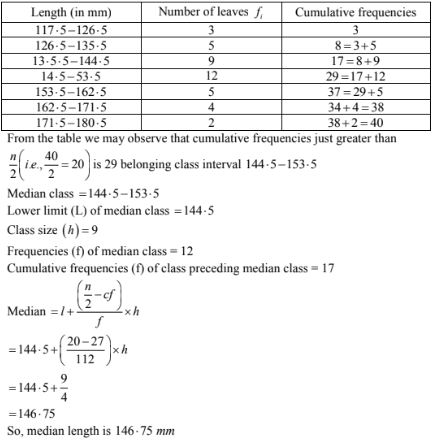18. The following table gives the distribution of the life time fo 400 neon lamps:
 Lite time(in hours) Number of lamps (1500 – 2000) 14 2000 – 2500 56 2500 – 3000 60 3000 – 3500 86 3500 – 4000 74 4000 – 4500 62 4500 – 5000 48

Find the median life.

Solution

We can find cumulative frequencies with their respective class intervals as below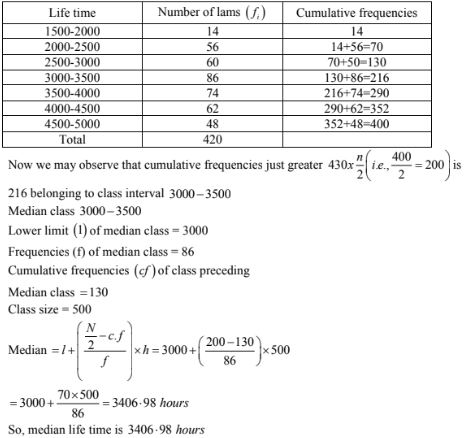19. The distribution below gives the weight of 30 students in a class. Find the median weight f students:
 Weight (in kg): 40 – 45 45 – 50 50 – 55 55 – 60 60 – 65 65 – 70 70 – 75 No. of students 2 3 8 6 6 3 2

Solution